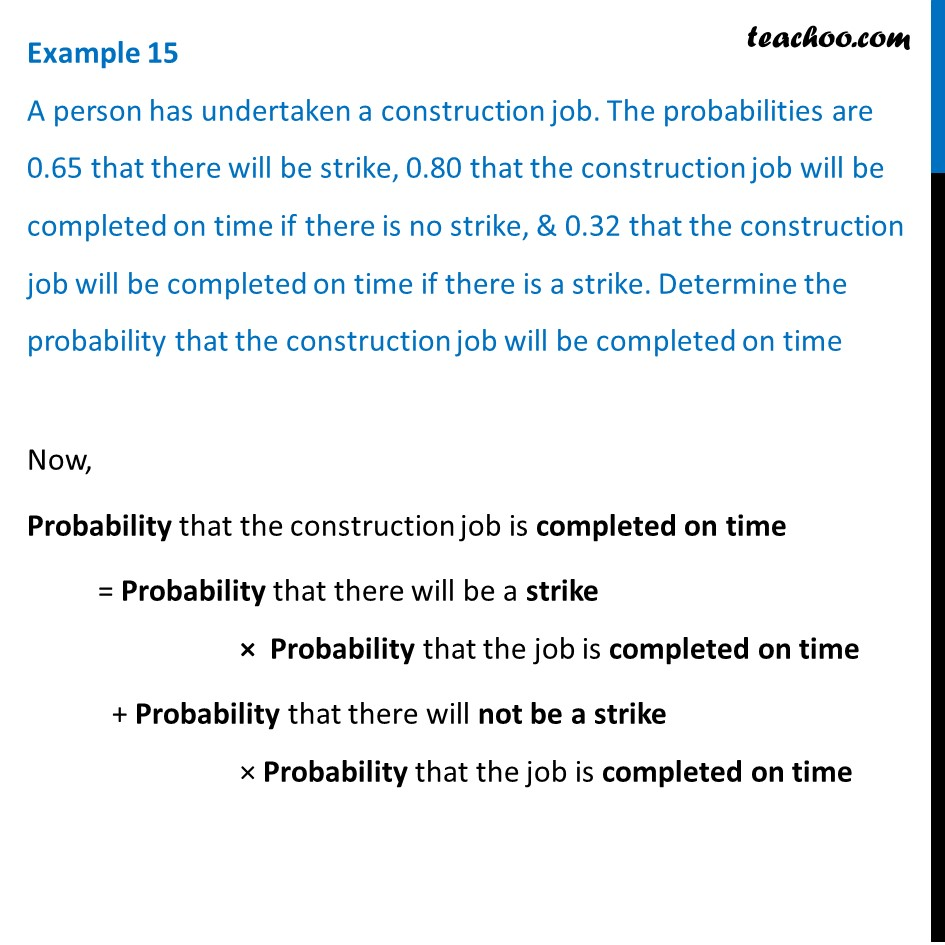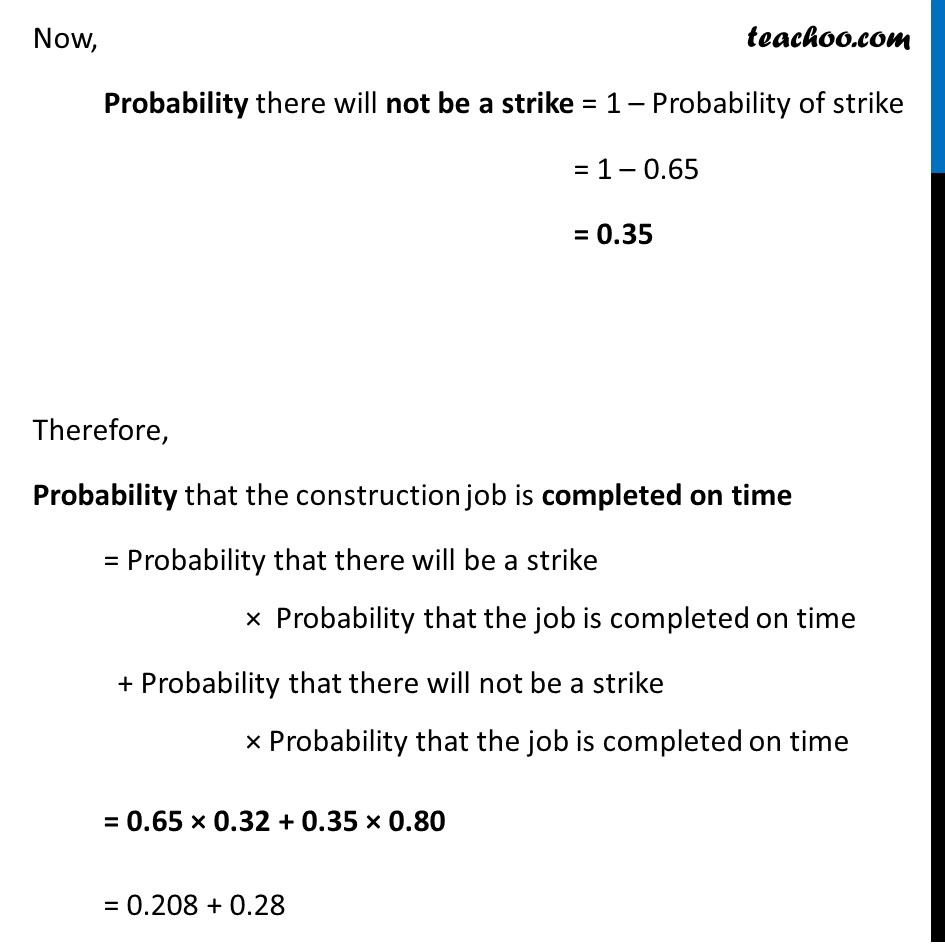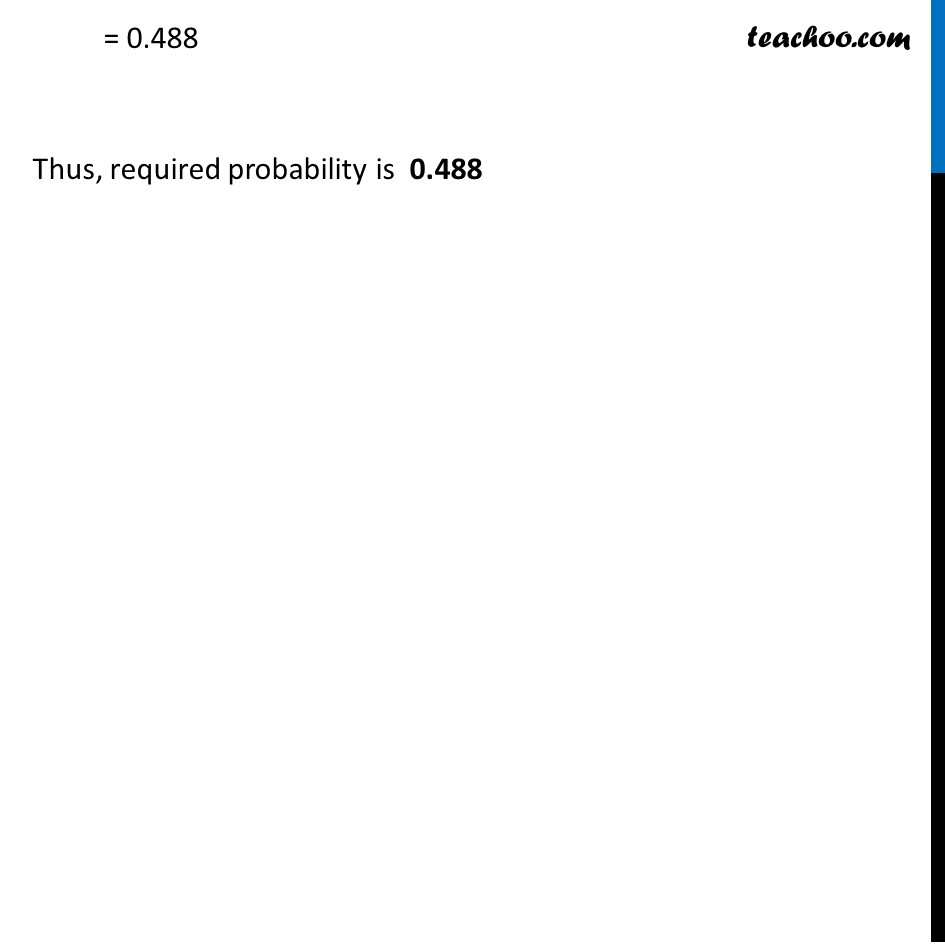Theorem of total probability

Chapter 13 Class 12 Probability
Concept wiseLearn in your speed, with individual attention - Teachoo Maths 1-on-1 Class

### Transcript

Example 15 A person has undertaken a construction job. The probabilities are 0.65 that there will be strike, 0.80 that the construction job will be completed on time if there is no strike, & 0.32 that the construction job will be completed on time if there is a strike. Determine the probability that the construction job will be completed on time Now, Probability that the construction job is completed on time = Probability that there will be a strike × Probability that the job is completed on time + Probability that there will not be a strike × Probability that the job is completed on time Now, Probability there will not be a strike = 1 – Probability of strike = 1 – 0.65 = 0.35 Therefore, Probability that the construction job is completed on time = Probability that there will be a strike × Probability that the job is completed on time + Probability that there will not be a strike × Probability that the job is completed on time = 0.65 × 0.32 + 0.35 × 0.80 = 0.208 + 0.28 = 0.488 Thus, required probability is 0.488